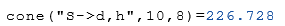# Class Cone

Calculation of the properties of cones

## Description

The class Cone calculates properties of a cone.

The default argument is the radius and the height. This means that the radius $$r$$ and the height $$h$$ can be passed as arguments without a command string (see example below).

## Property

The following properties can be queried as a result or passed as an argument.

#### Description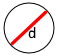d Base diameter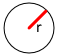r Base radius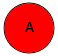A Base area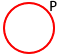P Base perimeter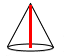h Cone height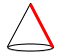L Surface height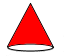Sl Surface without base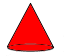S Total sureface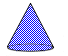V Volume

## Syntax

Object = Cone ("args names", arguments)

Value = Cone ("get –>args names", arguments)

## Examples

The default arguments are a radius of 5 and a height of 8.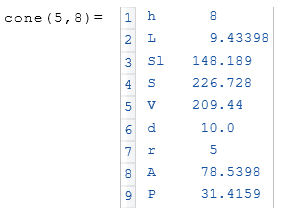From an initialized object $$a$$ only the volume $$V$$ is displayed.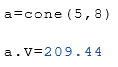Calculated from a diameter of 10 and a height of 8, the surface of the cone is calculated.